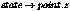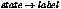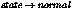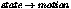Material shaders are the primary type of shaders. All materials defined in the scene must at least define a material shader. Materials may also define other types of shaders, such as shadow, volume, photon, and environment shaders, which are optional and of secondary importance.

When mental ray casts a visible ray, such as those cast by the camera (called primary rays) or those that are cast for reflections and refractions (collectively called secondary rays), mental ray determines the next object in the scene that is hit by that ray. This process is called intersection testing. For example, when a primary ray cast from the camera through the viewing plane's pixel (100,100) intersects with a yellow sphere, pixel (100, 100) in the output image will be painted yellow. (The actual process is slightly complicated by supersampling and filtering, which can cause more than one primary ray to contribute to a pixel.)

The core of mental ray has no concept of "yellow." This color is computed by the material shader attached to the sphere that was hit by the ray. mental ray records general information about the sphere object, such as point of intersection, normal vector, transformation matrix etc. in a data structure called the state, and calls the material shader attached to the object. More precisely, the material shader, along with its parameters (called shader parameters), is part of the material, which is attached to or inherited by the polygon or surface that forms the part of the object that was hit by the ray. Objects are usually built from multiple polygons and/or surfaces, each of which may have a different material.

The material shader uses the values provided by mental ray in the state and the variables provided by the .mi file in the shader parameters to calculate the color of the object, and returns that color. In the above example, the material shader would return the color yellow. mental ray stores this color in its internal sample list, which later gets filtered to compute frame buffer pixels, and then casts the next primary ray. Note that if the material shader has a bug that causes it to return infinity or NaN (Not a Number) in the result color, the infinity or NaN is stored as 1.0 in color frame buffers. This results in white pixels in the rendered image. This is true for subshaders such as texture shaders also.

With an appropriate output statement (see page output), mental ray computes depth, label, normal-vector, and motion vector frame buffers in addition to the standard color frame buffer, and up to eight user frame buffers defined with frame buffer statements in the options block. The color returned by the first-generation material shader is stored in the color frame buffer (unless a lens shader exists; lens shaders also have the option of modifying colors). The material shader can control what gets stored in the depth, label, normal-vector, and motion-vector frame buffers by storing appropriate values into,,, and, respectively. It can also store data in the user frame buffers with an appropriate call to mi_fb_put. Depth is the negative Z coordinate in camera space.

Material shaders normally do quite complicated computations to arrive at the final color of a point on the object:

• The shader parameters usually include constant ambient, diffuse, and specular colors and other parameters such as transparency, and possibly optional textures that need to be evaluated to compute the actual values at the intersection point. If textures are present, texture shaders are called by using one of the lookup functions provided by mental ray. Alternatively, shader assignment may be used for texturing. Texture shaders are discussed in the next section.
• The illumination computation sums up the contribution from various light sources listed in the shader parameters. To obtain the amount of light arriving from a light source, a light shader is called by calling a light trace or sample function provided by mental ray. Light shaders are discussed in a separate section below. After the illumination computation is finished, the ambient, diffuse, and specular colors have been combined into a single material color (assuming a more conventional material shader).

• If the material is reflective, transparent, or using refraction, as indicated by appropriate shader parameters, the shader must cast secondary rays and apply the result to the material color calculated in the previous step. (Transparency is a variation of refractive transparency where the ray continues in the same direction, while refraction rays may alter the direction based on an index of refraction.) Secondary rays, like primary rays, cause mental ray to do intersection testing and call another material shader if the intersection test hit an object. For this reason, material shaders must be reentrant. In particular, a secondary refraction or transparency ray will hit the back side of the same object if face both is set in the options and the object is a closed volume.

Note that the shader parameters of a material shader are under no obligation to define and use classical parameters like ambient, diffuse, and specular color and reflection and refraction parameters. Here is the source code of the mib_illum_phong shader in the standard base shader library:

```    #include <stdio.h>
#include <stdlib.h>         /* for abs */
#include <float.h>          /* for FLT_MAX */
#include <math.h>
#include <string.h>
#include <assert.h>

struct mib_illum_phong {
miColor         ambience;       /* ambient color multiplier */
miColor         ambient;        /* ambient color */
miColor         diffuse;        /* diffuse color */
miColor         specular;       /* specular color */
miScalar        exponent;       /* shinyness */
int             mode;           /* light mode: 0..2 */
int             i_light;        /* index of first light */
int             n_light;        /* number of lights */
miTag           light;       /* list of lights */
};

DLLEXPORT int mib_illum_phong_version(void) {return(2);}

DLLEXPORT miBoolean mib_illum_phong(
miColor         *result,
miState         *state,
struct mib_illum_phong *paras)
{
miColor         *ambi, *diff, *spec;
miTag           *light;         /* tag of light instance */
int             n_l;            /* number of light sources */
int             i_l;            /* offset of light sources */
int             m;              /* light mode: 0=all, 1=incl, 2=excl */
int             n;              /* light counter */
int             samples;        /* # of samples taken */
miColor         color;          /* color from light source */
miColor         sum;            /* summed sample colors */
miVector        dir;            /* direction towards light */
miScalar        dot_nl;         /* dot prod of normal and dir */
miScalar        expo;           /* Phong exponent (cosine power) */
miScalar        s;              /* amount of specular reflection */

ambi =  mi_eval_color(&paras->ambient);
diff =  mi_eval_color(&paras->diffuse);
spec =  mi_eval_color(&paras->specular);
expo = *mi_eval_scalar(&paras->exponent);
m    = *mi_eval_integer(&paras->mode);

*result    = *mi_eval_color(&paras->ambience);  /* ambient term */
result->r *= ambi->r;
result->g *= ambi->g;
result->b *= ambi->b;

n_l   = *mi_eval_integer(&paras->n_light);
i_l   = *mi_eval_integer(&paras->i_light);
light =  mi_eval_tag(paras->light) + i_l;

if (m == 1)             /* modify light list (inclusive mode) */
mi_inclusive_lightlist(&n_l, &light, state);
else if (m == 2)        /* modify light list (exclusive mode) */
mi_exclusive_lightlist(&n_l, &light, state);

/* Loop over all light sources */
for (n=0; n < n_l; n++, light++) {
sum.r = sum.g = sum.b = 0;
samples = 0;
while (mi_sample_light(&color, &dir, &dot_nl, state,
*light, &samples)) {
/* Lambert's cosine law */
sum.r += dot_nl * diff->r * color.r;
sum.g += dot_nl * diff->g * color.g;
sum.b += dot_nl * diff->b * color.b;

/* Phong's cosine power */
s = mi_phong_specular(expo, state, &dir);
if (s > 0.0) {
sum.r += s * spec->r * color.r;
sum.g += s * spec->g * color.g;
sum.b += s * spec->b * color.b;
}
}
if (samples) {
result->r += sum.r / samples;
result->g += sum.g / samples;
result->b += sum.b / samples;
}
}

/* add contribution from indirect illumination (caustics) */
result->r += color.r * diff->r;
result->g += color.g * diff->g;
result->b += color.b * diff->b;
result->a  = 1;
return(miTRUE);
}
```

(From now on, code examples will omit #include statements and version functions. For information on writing and using a corresponding .mi language declaration, see the shader declaration chapter on page declaration, or simply look at the base.mi file that comes with mental ray.)

This shader first evaluates all its parameters because there are no paths through the code that do not require some parameters. This shader is concerned exclusively with computing a BRDF (bidirectional reflectance distribution function, here Phong) but not with reflections, refractions, transparency, textures, etc. The BRDF is implemented as a loop over all lights. Each light is sampled with an inner sample loop implemented with mi_sample_light, which takes care of area light sources that require multiple samples. The contributions of each sample are summed, which produces the direct illumination component. Indirect illumination, computed by mi_compute_irradiance, is added at the end.

Reflection and refraction can be added by another material shader that handles only refraction, and works with a BRDF material like the one above by assigning that shader to one of its inputs (here, the input parameter). The following shader handles transparency (defined as transmission with an index of refraction of 1.0, refraction (an index other than 1.0), and total internal reflection. Total internal reflection happens for rays that hit the surface at a grazing angle; it is the reason why one sees the near part of the bottom of a pool, but at greater distances the water surface reflects the sky.

```    struct mr {
miColor         input;
miColor         refract;
miScalar        ior;
};

DLLEXPORT miBoolean mib_refract(
miColor         *result,
miState         *state,
struct mr       *paras)
{
miColor         *refract = mi_eval_color(&paras->refract);
miColor         inp;
miVector        dir;
miScalar        ior;

if (refract->r == 0.0 && refract->g == 0.0 &&
refract->b == 0.0 && refract->a == 0.0)
*result = *mi_eval_color(&paras->input);
else {
ior = *mi_eval_scalar(&paras->ior);
if (ior == 0.0 || ior == 1.0)
mi_trace_transparent(result, state);
else {
if (mi_refraction_dir(&dir, state, 1.0, ior))
mi_trace_refraction(result, state, &dir);
else {  /* total internal reflection */
mi_reflection_dir(&dir, state);
mi_trace_reflection(result, state, &dir);
}
}
if (refract->r != 1.0 || refract->g != 1.0 ||
refract->b != 1.0 || refract->a != 1.0) {
inp = *mi_eval_color(&paras->input);
result->r = result->r * refract->r +
inp.r * (1.0 - refract->r);
result->g = result->g * refract->g +
inp.g * (1.0 - refract->g);
result->b = result->b * refract->b +
inp.b * (1.0 - refract->b);
result->a = result->a * refract->a +
inp.a * (1.0 - refract->a);
}
}
return(miTRUE);
}
```

Note that this shader evaluates its input parameter only if the value is actually needed, to avoid wasting time computing a BRDF whose result is discarded. The ability to ask for parameter values explicitly is the reason for the mi_eval facility in mental ray. This shader makes this even more obvious:

```    struct mt {
miColor front;
miColor back;
};

DLLEXPORT miBoolean mib_twosided(
miColor         *result,
miState         *state,
struct mt       *paras)
{
if (state->inv_normal)
*result = *mi_eval_color(&paras->back);
else
*result = *mi_eval_color(&paras->front);
return(miTRUE);
}
```

This shader can be used as a material shader that applies different materials to the front and back side of an object. Front and back are determined by the direction of the normal vector. The two materials are simply assigned to the front and back parameters. Clearly, it would be very inefficient if this shader evaluated both its inputs.

Bump mapping is another common function of material shaders. It involves altering the normal vector before computing the BRDF, because the normal determines the surface orientation and is essential to BRDF computation. Here is a bump map shader that computes and returns a new normal vector:

```    struct mib_bump_map_simple {
miVector        u;
miVector        v;
miVector        coord;
miVector        step;
miScalar        factor;
miTag           tex;
};

DLLEXPORT miBoolean mib_bump_map_simple(
miVector        *result,
miState         *state,
struct mib_bump_map_simple *paras)
{
miTag           tex     = *mi_eval_tag    (&paras->tex);
miVector        coord   = *mi_eval_vector (&paras->coord);
miVector        step    = *mi_eval_vector (&paras->step);
miVector        u       = *mi_eval_vector (&paras->u);
miVector        v       = *mi_eval_vector (&paras->v);
miScalar        factor  = *mi_eval_scalar (&paras->factor);
miVector        coord_u, coord_v;
miScalar        val, val_u, val_v;
miColor         color;

coord_u.x = coord.x + (step.x ? step.x : 0.001);
coord_u.y = coord.y;
coord_u.z = coord.z;
coord_v.x = coord.x;
coord_v.y = coord.y + (step.y ? step.y : 0.001);
coord_v.z = coord.z;
if (!tex || !mi_lookup_color_texture(&color, state, tex, &coord)) {
*result = state->normal;
return(miFALSE);
}
val = (color.r + color.g + color.b) / 3;
mi_flush_cache(state);
val_u = mi_lookup_color_texture(&color, state, tex, &coord_u)
? (color.r + color.g + color.b) / 3 : val;

mi_flush_cache(state);
val_v = mi_lookup_color_texture(&color, state, tex, &coord_v)
? (color.r + color.g + color.b) / 3 : val;
val_u -= val;
val_v -= val;
state->normal.x += factor * (u.x * val_u + v.x * val_v);
state->normal.y += factor * (u.y * val_u + v.y * val_v);
state->normal.z += factor * (u.z * val_u + v.z * val_v);
mi_vector_normalize(&state->normal);
*result = state->normal;
return(miTRUE);
}
```

This is a simplified version of the mib_bump_map shader in the base library that lacks some nonessential projection features. It looks up a texture map at the current point and one point above and to the side each, and perturbs the normal vector depending on the differences between these sampling points. Here a shader assignment of the texture would not work because that would generate the same result three times.

Moreover, it is necessary to call mi_flush_cache to turn off mental ray's result caching, which normally prevents a shader to be called multiple times if it is evaluated multiple times. This happens frequently, for example when a texture shader is assigned to both the ambient and diffuse parameters of a BRDF material shader - obviously it would be inefficient to compute the same texture twice when the BRDF shader evaluates both parameters, so the second evaluation simply causes mental ray to return the cached result from the first evaluation. Here this would defeat the purpose, so the cache must be cleared explicitly.

The following shader is a variation on the first. Instead of returning a vector, it leaves the return value unchanged, but still modifies thestate variable that BRDF functions use for illumination calculations:

```    DLLEXPORT miBoolean mib_passthrough_bump_map_simple(
miColor         *result,
miState         *state,
struct mib_bump_map_simple *paras)
{
miVector        dummy;

return(mib_bump_map_simple(&dummy, state, paras));
}
```

This way, the shader can simply be inserted in a shader list, as in

```    material "mtl"
"mib_passthrough_bump_map_simple" ()
"mib_illum_phong" (...)
end material
```

mental ray will first call the bump passthrough shader, which perturbs, and then the Phong shader, which uses the normal. Since the passthrough bump shader does not alter its result, the end result is the BRDF.

Texturing can be handled by calling shader API functions such as mi_lookup_color_texture in the material (or other) shaders, but it is easier to simply assign a texture shader to one of the BRDF color inputs, especially ambient and diffuse in the Phong example above.

Hair material shaders work like normal material shaders, with a few changes in the state:

• bary contains the parameter value along the width of the hair, with 0 at the left edge and 1 at the right edge, with respect to the direction going from the root of the hair to the tip, and in a plane perpendicular to the incoming ray.
• bary contains the parameter value along the length of the hair, with 0 at the first vertex defined, and 1 at the last.
• normal_geom is the tangent of the first segment of the hair. This is an approximation to the normal at the "scalp", and can be useful for certain shading models.
• normal is the interpolated normal facing the ray direction.
• derivs is the interpolated tangent at the point of intersection.
• tex_list contains texture scalars in the order .x, .y, .z, .x, and so on. This is equivalent to the way other geometry stores texture data, except that hair uses scalars, not vectors, and their number does not have to be a multiple of three. It is often useful to recast tex_list to a miScalar pointer.

Hair material shaders should not normally assume that hair consists of geometrical cylinders that can be shaded normally, complete with a terminator and all. Hair is too fine for shading around the circumference; any attempt to place highlights on just one side of the hair may cause aliasing. The base shader library shipped with mental ray contains a special hair material shader mib_illum_hair for this purpose.

Copyright © 1986-2007 by mental images GmbH